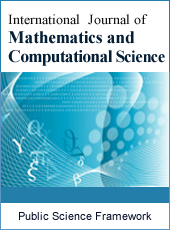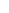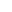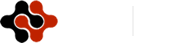International Journal of Mathematics and Computational Science
Articles Information
International Journal of Mathematics and Computational Science, Vol.2, No.2, Apr. 2016, Pub. Date: May 12, 2016
Construction of Analytic Solution for Coupled Cubic Nonlinear Systems Using Homotopy Analysis Method
 J. M. Guo, College of Mathematics, Physics and Information Engineering, Zhejiang Normal University, Jinhua, Zhejiang, P. R. China.
 Y. H. Qian, College of Mathematics, Physics and Information Engineering, Zhejiang Normal University, Jinhua, Zhejiang, P. R. China.
 S. P. Chen, College of Mathematics, Xiamen University of Technology, Xiamen, P. R. China.
In this paper, the existence solution of initial value problem for coupled cubic nonlinear systems is proved at first. Then by using the homotopy analysis method (HAM), an analytical approximation of those systems can be obtained. It is full of freedom to choose a set of base functions when using the HAM, and as the set of base functions is chosen differently, the analytical approximation solutions which will have some different effect. Therefore, it is an interesting and meaningful task to get a more efficient analytical approximation by a better set of base functions. Furtherly, by combining the HAM with padé approximation, the result can be obtained on broader region of convergence. To illustrate the accuracy of the present method, the solutions obtained in this paper are compared with those of Runge-Kutta method, which shows the HAM is effective and feasible.
Initial Value Problem, Coupled Cubic Nonlinear Systems, the Homotopy Analysis Method, Homotopy Padé Approximation
 S. M. Fang and B. L. Guo , Existence of time periodic solutions for a damped generalized coupled nonlinear wave equations, Applied Mathematics and Mechanics , 24, p673-683, 2003.
 J. X. Liu , X. Q. Wang, and B. Wang, General solution for the coupled equations of transversely isotropic magnetoelectroelastic solids, Applied Mathematics and Mechanics, 24, p774-781, 2003.
 A. S. J. Al-Saif and Z. Y. Zhu, Application of mixed differential quadrature method for solving the coupled two-dimensional incompressible Navier-stokes equation and heat equation, Journal of Shanghai University, 7, p343-351, 2003.
 L. Bougoffa and S. Bougouffa, Adomian method for solving some coupled systems of two equations, Applied Mathematics and Computation, 177, p553-560, 2006.
 S. J. Liao, Beyond perturbation: Introduction to the homotopy analysis method, Beijing Science Press, 2006.
 S. J. Liao and A. T. Chwang, Application of homotopy analysis method in nonlinear oscillation, ASME Journal of Applied Mechanics, 65, p914-922, 1998.
 S. J. Liao, An analytic approximate approach for free oscillations of self-excited systems, International Journal of Non-Linear Mechanics, 39, p271-280, 2004.
 S. J. Liao, On the homotopy analysis method for nonlinear problems, Applied Mathematics and Computation, 147, p499-513, 2004.
 H. Xu, An explicit analytic solution for free convection about a vertical flat plate embedded in a porous medium by means of homotopy analysis method, Applied Mathematics and Computation, 158, p33-443, 2004.
 F. M. Allan and M. I. Syam, On the analytic solutions of the nonhomogeneous Blasius problem, Journal of Computational and Applied Mechanics, 182, p362-371, 2005.
 S. Abbasbandy, The application of homotopy analysis method to nonlinear equations arising in heat transfer, Physics Letters A, 360, p109-113, 2006.
 T. Hayat and M. Sajid, On analytic solution for thin flow of a fourth grade fluid down a vertical cylinder, Physics Letters A, 361, p316-322, 2007.
 T. Hayat, N. Ahmed, M. Sajid and S. Asghar, On the MHD flow of a second grade fluid in aporous channel, Computers and Mathematics with Applications, 54, p407-414, 2007.
 T. Hayat, F. Shahzad and M. Ayub, Analytical solution for the steady flow of the third grade fluid in a porous half space, Applied Mathematical Modelling, 31, p424-2432, 2007.
 L. Song and H. Zhang, Application of homotopy analysis method to fractional KdV-Burgers-Kuramoto equation, Physics Letters A, 367, p88-94, 2007.
 M. Inc, On exact solution of Lapalece equation with Dirichlet and Neumann boundary conditions by the homotopy analysis method, Physics Letters A 365, p412-415, 2007.
 Y. J. Li, B. T. Nohara and S. J. Liao, Series solutions of coupled van der Pol equation by means of homotopy analysis method, Journal of Mathematical Physics, 51, Article No. 063517, 2010.
 W. Zhang, Y. H. Qian, M. H. Yao and S. K. Lai, Periodic solutions of multi-degree-of-freedom strongly nonlinear coupled van der Pol oscillators by homotopy analysis method, Acta Mechanica, 217, p269-285, 2011.
 R. Cobiaga, and W. Reartes, Search for Periodic Orbits in Delay Differential Equations, International Journal of Bifurcation and Chaos, 24, Article No. 1450084, 2014.
 P. Wang and D. Q. Lu, Homotopy-based analytical approximation to nonlinear short-crested waves in a fluid of finite depth, Journal of Hydrodynamics, 27, p321-331, 2015.
 S. J. Liao, An optimal homotopy-analysis approach for strongly nonlinear differential equations, Communication in Nonlinear Sciences and Numerical Simulation, 15, p2003-2016, 2010.
 Z. Niu and C. Wang, A one-step optimal homotopy analysis method for nonlinear differential equations, Communication in Nonlinear Sciences and Numerical Simulation, 15, p2026-2036, 2010.
 T. pirbodaghil and S. Hoseini, Nonlinear free vibration of a symmetrically conservative two-mass system with cubic nonlinearity, Journal of Computational and Nonlinear Dynamics 5, Article No. 011006, 2010.
 G. López and L. J. M. Ceniceros, Fourier-Padé approximants for Nikishin systems, Constructive Approximation, 30, p53-69, 2009.
 M. F. Wang and F. T. K. Au, On the precise integration methods based on Padé approximations, Computers and Structures, 87, p380-390, 2009.
 K. Q. Le, Complex Padé approximant operators for wide-angle beam propagation, Optics Communications, 282, p1252-1254, 2009.
 Z. B. Li, J. K. Tang and P. Cai, A generalized Padé approximation method of solving homoclinic and heteroclinic orbits of strongly nonlinear autonomous oscillators, Journal of Sound and Vibration, 332, p5508–5522, 2013.
 G. C. Zhao, J. R. Kong, L. P. Song, X. Du, L. Shan and H. Zhao, Analytical solutions of velocity and temperature in laminar boundary layer over a flat plate with wall injection flow using HPM-Padé method, Journal of Aerospace Power, 30, p1794-1801, 2015.
 H. Vazquez-Leal, J. Rashidinia, L. Hernandez-Martinez and H. Daei-Kasmaei, A comparison of HPM, NDHPM, Picard and Picard-Padé methods for solving Michaelis-Menten equation, Journal of King Saud University-Science, 27, p7-14, 2015.
 W. Walter, Ordinary Differential Equations, World Publishing Corporation Press, 2003.ISSN Print: 2381-7011
ISSN Online: 2381-702X
Current Issue:
Vol. 5, Issue 2, June Submit a Manuscript Join Editorial Board Join Reviewer Team
 About This Journal All Issues Open Access Indexing Payment Information Author Guidelines Review Process Publication Ethics Editorial Board Peer Reviewers600 ATLANTIC AVE, BOSTON,
MA 02210, USA+001-6179630233
JournalsJournal ListsAuthor GuidelinesPayment InformationSubmit a ManuscriptJoin as Editorial MembersJoin as ReviewersPrivacy NoticeTerms of ConditionsHelp Center